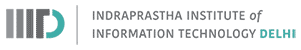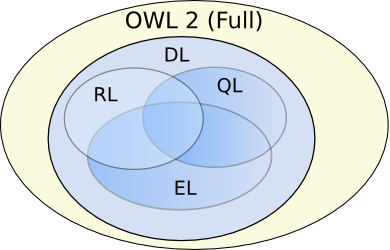## Description Logic Reasoning

Winter 2019
Instructor: Raghava Mutharaju
IIIT-Delhi### Description Logic Reasoning

• Reasoning is the process of deriving facts that are not explicitly expressed in the ontology
• Reasoning makes implicit facts explicit
• Logical consequences are drawn from the axioms in the ontology

### Description Logic Reasoning

• Axioms before reasoning
• {john} $\sqsubseteq$ USCitizen
• USCitizen $\sqsubseteq \exists$hasPassport.USPassport
• $\exists$hasPassport.USPassport $\sqsubseteq$ EUVisaNotRequired
• Logical consequences after reasoning
• USCitizen $\sqsubseteq$ EUVisaNotRequired
• {john} $\sqsubseteq$ EUVisaNotRequired

### Purpose of DL Reasoning

1. Design and maintain high quality ontologies
• Consistency: reasoner helps in determining whether an ontology is consistent or not
• Minimally redundant: reasoner checks for unintended synonyms
2. Answer queries over ontology classes and instances
• Find more general/specific classes
• Retrieve individuals of a class

### Expressivity and Complexity Trade-off

#### OWL 2 ProfilesImage source: https://goo.gl/HDvOZe

#### Reasoning Complexity

• OWL 2 Full: Undecidable
• OWL 2 DL: N2EXPTIME (22n in the size of input)
• OWL 2 EL, OWL 2 RL, OWL 2 QL: Polynomial time

### Types of Reasoning

• Forward chaining
• Start with the axioms and apply inference rules repeatedly until no more new axioms can be derived
• Saves time during querying but ends up using more space
• Backward chaining
• Start with a query and apply inference rules that are needed to only answer the query
• Saves space but increases the querying time

### Important Reasoning Tasks

• Consistency of the knowledge base (ontology in our case)
• Class level consistency (is class C empty, $C \equiv \bot$?)
• Class inclusion (is C $\sqsubseteq$ D?)
• Class equivalence (is C $\equiv$ D?)
• Class disjointness (is C $\sqcap$ D $\equiv \bot$?)
• Class membership (is C(a)?)
• Instance retrieval (list all the instances of class C)

### Reasoning Procedure

• Rule based reasoning
• Tableaux algorithm

### Rule based reasoning

• If $X \sqsubseteq A$ and $A \sqsubseteq B$ then $X \sqsubseteq B$
• If $X \sqsubseteq A_1$, $X \sqsubseteq A_2$, $\ldots$, $X \sqsubseteq A_n$, and $A_1 \sqcap A_2 \sqcap \ldots \sqcap A_n \sqsubseteq B$ then $X \sqsubseteq B$
• Examples
• Mother $\sqsubseteq$ Female, Mother $\sqsubseteq$ Parent, and Female $\sqcap$ Parent $\sqsubseteq$ Human
• Is Mother a Human?

### Tableaux Algorithm

• They are used to test satisfiability (consistency)
• A tree (there can be loops) is built with nodes consisting of class expressions to be evaluated
• Edges are labelled with the relationships between classes
• Nodes are expanded by applying the tableaux rules
• Tree expansion stops when either no more rules can be applied or a clash occurs (for eg: $A \sqcap \neg A$)
• Apply Negation Normal Form on the given Knowledge Base K
• Apply tableaux rules on the axioms
• Check for termination
• If there is a contradiction in the tableaux, then it is unsatisfiable

### Negation Normal Form

• It simplifies the given knowledge base
• It is a syntactic transformation
• Initial steps
• Replace C $\equiv$ D with C $\sqsubseteq$ D and D $\sqsubseteq$ C
• Replace C $\sqsubseteq$ D with $\neg$C $\sqcup$ D
• Apply the NNF rules exhaustively
• In NNF(K), negation occurs only directly in front of atomic classes
• NNF(C) = C, if C is a class name
• NNF($\neg$C) = $\neg$C, if C is a class name
• NNF($\neg\neg$C) = NNF(C)
• NNF(C $\sqcup$ D) = NNF(C) $\sqcup$ NNF(D)
• NNF(C $\sqcap$ D) = NNF(C) $\sqcap$ NNF(D)
• NNF($\neg$(C $\sqcup$ D)) = NNF($\neg$C) $\sqcap$ NNF($\neg$D)
• NNF($\neg$(C $\sqcap$ D)) = NNF($\neg$C) $\sqcup$ NNF($\neg$D)
• NNF($\forall$R.C) = $\forall$R.NNF(C)
• NNF($\exists$R.C) = $\exists$R.NNF(C)
• NNF($\neg\forall$R.C) = $\exists$R.NNF($\neg$C)
• NNF($\neg\exists$R.C) = $\forall$R.NNF($\neg$C)
• Transform the axiom below to NNF
• P $\sqsubseteq$ (E $\sqcap$ M) $\sqcup$ $\neg$($\neg$E $\sqcup$ D)
• Step-1: $\neg$P $\sqcup$ ((E $\sqcap$ M) $\sqcup$ $\neg$($\neg$E $\sqcup$ D))
• Step-2: $\neg$P $\sqcup$ ((E $\sqcap$ M) $\sqcup$ (E $\sqcap$ $\neg$D))

### Tableaux Algorithm

• Input: Knowledge Base, K = TBox + ABox, in NNF
• Output: Whether or not K is satisfiable

• A tableau is a directed labelled graph
• Nodes are individuals or (new) variable names
• Nodes x are labelled with sets L(x) of class expressions
• Edges <x,y> are labelled with sets L(<x,y>) of role names

#### Initialization

• Make a node for every individual in the ABox
• Every node is labelled with the corresponding class names from the ABox
• There is an edge, labelled with R, between a and b, if R(a,b) is in the ABox
• If there is no ABox, the initial tableau consists of a node x with empty label

#### Initialization

• Build an initial tableau for the following knowledge base
• K = {A(a),($\exists$R.B)(a),R(a,b),R(a,c),S(b,b),(A$\sqcup$B)(c),$\neg$A$\sqcup$($\forall$S.B)}

#### Naive $ALC$ Tableaux Rules

• $\sqcap$-rule: If C $\sqcap$ D $\in$ L(x) and {C,D} $\not\subseteq$ L(x), then set L(x) $\leftarrow$ {C,D}
• $\sqcup$-rule: If C $\sqcup$ D $\in$ L(x) and {C,D} $\cap$ L(x) = $\emptyset$, then set L(x) $\leftarrow$ C or L(x) $\leftarrow$ D
• $\exists$-rule: If $\exists$R.C $\in$ L(x) and there is no y with R $\in$ L(x,y) and C $\in$ L(y), then
1. add a new node with label y (where y is a new node label),
2. set L(x,y) = {R}, and
3. set L(y) = {C}
• $\forall$-rule: If $\forall$R.C $\in$ L(x) and there is a node y with R $\in$ L(x,y) and C $\not\in$ L(y), then set L(y) $\leftarrow$ C
• TBox-rule: If C is a TBox statement and C $\not\in$ L(x), then set L(x) $\leftarrow$ C

#### Examples

• K = {C(a), C $\sqsubseteq$ $\exists$R.D, D $\sqsubseteq$ E}. Is ($\exists$R.E)(a) a logical consequence?
• K = {Human $\sqsubseteq$ $\exists$hasParent.Human, Bird(tweety)}. Is tweety not Human?

• Tableau constructed on whiteboard

#### Examples

• Try the following
• Human $\sqsubseteq$ $\exists$hasParent.Human
• Orphan $\sqsubseteq$ Human $\sqcap$ $\forall$hasParent.$\neg$Alive
• Orphan(harrypotter)
• hasParent(harrypotter, jamespotter)

• Is $\neg$Alive(jamespotter), a logical consequence?

#### Blocking

• K = {$\exists$R.$\top$, $\top$($a_1$)}. Check the satisfiability of K
• Does the Tableau for K terminate?

• Tableau constructed on whiteboard

• Observation: Node x has the same properties of node $a_1$. So "reuse" $a_1$

#### Blocking

• Terms: Consider L(x,y) $\neq$ $\emptyset$. Let's say L(x,y) = {R}
• Successor: y is the successor (R-successor) of x
• Predecessor: x is the predecessor (R-predecessor) of y
• Ancestor: Every predecessor of y, which is not an individual, is an ancestor of y. Every predecessor of an ancestor of y, which is not an individual, is also an ancestor of y

#### Blocking

• A node with a label x is blocked by a node with label y if
• x is a variable but not an individual
• y is an ancestor of x, and L(x) $\subseteq$ L(y)
• or an ancestor of x is blocked

#### Naive $ALC$ Tableaux Rules (with blocking)

• $\sqcap$-rule: If C $\sqcap$ D $\in$ L(x) and {C,D} $\not\subseteq$ L(x), then set L(x) $\leftarrow$ {C,D}
• $\sqcup$-rule: If C $\sqcup$ D $\in$ L(x) and {C,D} $\cap$ L(x) = $\emptyset$, then set L(x) $\leftarrow$ C or L(x) $\leftarrow$ D
• $\exists$-rule: If $\exists$R.C $\in$ L(x) and there is no y with R $\in$ L(x,y) and C $\in$ L(y), then
1. add a new node with label y (where y is a new node label),
2. set L(x,y) = {R}, and
3. set L(y) = {C}
• $\forall$-rule: If $\forall$R.C $\in$ L(x) and there is a node y with R $\in$ L(x,y) and C $\not\in$ L(y), then set L(y) $\leftarrow$ C
• TBox-rule: If C is a TBox statement and C $\not\in$ L(x), then set L(x) $\leftarrow$ C
• Apply these rules only if x is not blocked

#### Blocking

• K = {$\exists$R.$\top$, $\top$($a_1$)}
• K = {Human $\sqsubseteq$ $\exists$hasParent.Human, Bird(tweety)}. Is tweety not Human?
• K = {hasChild(john,peter), hasChild(john,alex), Male(peter), Male(alex)}. Show that $\forall$hasChild.male(john) is not a logical consequence of K
• K = {Bird $\sqsubseteq$ Flies, Penguin $\sqsubseteq$ Bird, Penguin $\sqcap$ Bird $\sqsubseteq$ $\bot$, Penguin(tweety)}. Show K is unsatisfiable

• Tableau constructed on whiteboard

## References

1. Foundations of Semantic Web Technologies. Pascal Hitzler et. al. CRC Press.
2. OWL 2 Profiles. Boris Motik et. al. 2012